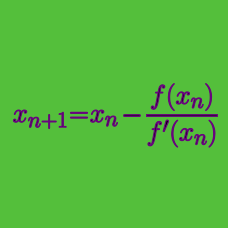Calculus

# Root Approximation: Level 3 Challenges

$\large \color{red}{3}x^{5} \color{blue}{=} \color{red}{1}x^{4} + \color{red}{4}x^{3} + \color{red}{1}x^{2} + \color{red}{5}x + \color{red}{9}$

The above polynomial has exactly one real root $$\alpha$$ . Find $$\lfloor 1000 \times \alpha \rfloor$$.

Notation: $$\lfloor x \rfloor$$ denotes the greatest integer smaller than or equal to $$x$$. This is known as the greatest integer function.

Consider this equation

$2^x=x^2$

There are 2 trivial solutions, namely $$x=2$$ and $$x=4$$

However, the equation is known to also have a negative solution. Find the negative solution.

Give your answer to 3 decimal places.

Find the value of $$a$$ to 3 significant figures, so that $$x$$ and $$y$$ are distinct real solutions to

$x+y=100\pi, \\ x^y = y^x = a.$

$\large x^y = y^x = 43$

If $$x$$ and $$y$$ are distinct positive real numbers satisfying the equation above, find $$\lfloor 100(x+y) \rfloor$$.

You are allowed to use computer assistance.

You might refer to Soumava's algorithm.

$f(x) = 9x^8 + 7x^6 + 5x^4 + 3x^2 - 1 = 0$

Which option is closest to the largest real root of the equation above?

×

Problem Loading...

Note Loading...

Set Loading...